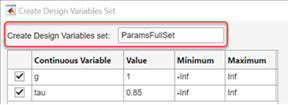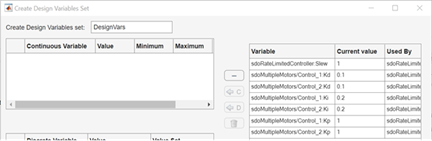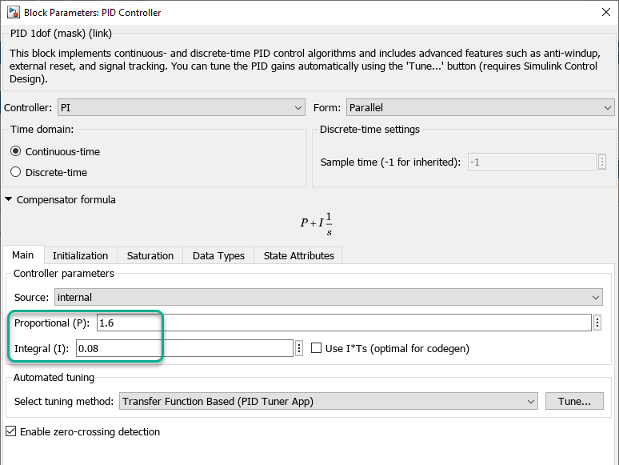## Specify Design Variables for Optimization

Response optimization is the process of varying the values of model parameters to make certain signals in the model satisfy design requirements. In addition to specifying these design requirements, such as signal bounds or reference values, you must also identify the model parameters that the optimizer can modify. These parameters form the design variables set for optimization.

Design variables in Simulink® Design Optimization™ software can take scalar, vector, or matrix values, or be specified as an expression that evaluates to such a value. Design variables can vary continuously, or take values restricted to a discrete, finite set. When you specify design variables at the command line or in the Response Optimizer app, you designate each variable as either continuous or discrete valued. For continuous variables, you can specify variable bounds for the optimization. For discrete variables, you specify the set of allowed values, which can be numeric or string values.

### Identify Variables to Optimize

To simplify the response optimization problem and save time and computing resources, it can be useful to focus your optimization on variables that most influence the response. Sensitivity analysis is one way to identify those variables. In the Response Optimizer app, you can access the Sensitivity Analyzer app by clickingSensitivity Analysis. In the Sensitivity Analyzer app, you can explore the response optimization design space, identify the variables that most influence the optimization problem, and compute initial values. Then, return to the Response Optimizer app and use the most sensitive variables for your optimization. For more information, see Identify Key Parameters for Estimation (GUI) and Use Sensitivity Analysis to Configure Estimation and Optimization.

### Specify Design Variables and Values

When you know which model parameters you want to tune, add them to a set of design variables in the Response Optimizer app as follows.

1. Click Design Variables Set and selectNew.The Create Design Variables Set dialog box opens. The table on the right side of the dialog box is populated with the model variables that are available to tune.Note

You can only optimize variables that are in use by the Simulink model. If the model variable you want to tune does not appear in the list, see Add Model Parameters as Variables for Optimization.

2. Add continuous variables, variables whose value can vary continuously over a range. In the table on the right side, select one or more variable names and clickC. The selected variables move to the Continuous Variable table on the left side of the dialog box.

Specify continuous-variable attributes such as the initial value and bounds on values the variables can take during optimization.

• Value — Initial value for optimization. By default, the initial value is the current value in the model. If you change the initial value in the table, click to update the values in the model.

• Minimum and Maximum — Lower and upper bounds on the value the variable can take during optimization, respectively. The defaults are `-Inf` and `Inf`.

Often, there are computational advantages in specifying finite bounds. Furthermore, it can be important to specify finite bounds to get meaningful results. For example, if a variable specifies a quantity that must be positive (such as the mass of a physical object), specify `0` as the absolute lower bound even if better information is unavailable.

• Scale — Scaling factor. During optimization, the design variables are scaled, or normalized, by dividing their current value by a scale value. The default value is the nearest power of 2 greater than the current value of the variable. Specifying a scaling factor can be useful when your variables are separated by several orders of magnitude.

3. Add discrete variables, variables whose values are restricted to specified finite sets of values. In the table on the right side, select one or more variable names and clickD. The selected variables move to the Discrete Variable table on the left side of the dialog box. Specify the current values and value sets (set of allowed values for optimization) for each variable. (since R2023a)

• Value — Initial value for optimization. By default, the initial value is the current value in the model. The initial value must be an element of the value set. If you change the initial value in the table, click to update the values in the model.

• Value Set — Vector of values that the variable can take during optimization. The default value is the current value of the variable. Edit this field to specify allowed values. Discrete variables are not limited to integer or evenly spaced values, and can be string values. For example, `[1.0,1.5,3.0,5.5]`, `1:10`, or `["off","low","high"]`. If you specify a value set that does not include the initial value, then the Value field updates to the first element of the value set.

Note

Optimizing discrete variables requires using the surrogate optimization method. To specify this method in the app, selectMore Options > Optimization. Then, in the Estimation Options dialog box, in the Optimization tab, in the Method menu, select `Surrogate optimization`. When you use this method, all continuous variables must have finite bounds.

4. Name the design variable set. Enter a name for the set in the Create Design Variables set field.5. Click to create the design variable set.

#### Tips for Configuring Design Variables Set

• The check box at the left side of each variable table indicates whether the variable is selected as a design variable in the set. Clear a checkbox to exclude the corresponding variable from the optimization but keep it in the set of design variables to use in a subsequent optimization.

• To find what block in the model contains a particular variable, select the variable and click Variable Detail.

• If a model parameter that you want to use for optimization is not available in the variable list, you might need to add a model variable for that parameter. See Add Model Parameters as Variables for Optimization.

• The continuous variables that you select must have a numeric value that uses the data type `double`. Discrete variables can take numeric or string values. If the value of a variable does not meet these conditions, use these techniques:

• To select a single element or a subset of a matrix or array variable, click Specify expression indexing if necessary.Enter an expression such as `myArray(2)`, which selects the second element of an array variable `myArray`.

After you type the expression, press the Enter key to add the variable to the list of model variables.

• To use a variable of a numeric data type other than `double`, convert the variable to a `Simulink.Parameter` object, which separates a parameter value from its data type. Set the `Value` property to a default `double` number, and use the `DataType` property to control the data type.

• To use the value of a `Simulink.Parameter` object, specify the `Value` property. Enter the expression `myParamObj.Value`.

• To use a field of a structure, enter `myStruct.PID.P1`. If you store the structure in a `Simulink.Parameter` object, enter `myStruct.Value.PID.P1`.

• To use one cell of a cell array, enter `myCells{3}`.

You cannot use mathematical expressions such as a + b. Sometimes, models have parameters that are not explicitly defined in the model itself. For example, a gain `k` could be defined in the MATLAB® workspace as `k = a + b`, where `a` and `b` are not defined in the model but `k` is used. To add these independent parameters, see Add Model Parameters as Variables for Optimization.

#### Variables in Referenced Models

If your model contains referenced models, you can select the referenced variables from the Create Design Variables Set dialog box. For example, in the illustration below, the first variable in the dialog box, `Slew`, is listed as `sdoRateLimitedController:Slew`. `sdoRateLimitedController` is the name of the referenced model with the variable `Slew`. The `Slew` variable has the same value for all instances of the `sdoRateLimitedController` model. In contrast, the variable `Kd` can have a different value for each instance of the referenced model containing it. For example, the second variable in the dialog box is listed as `sdoMultipleMotors/Control_1:Kd`. The upper-level model `sdoMultipleMotors` has block `Control_1`, which is a referenced model that has variable `Kd`. The value of this variable can be different than `Kd` in block `Control_2`, which is the third variable in the dialog box. To enable instance-specific values, `Kd` is specified as a model argument in the referenced model workspace.For a full example, see Design Optimization Tuning Parameters in Referenced Models (GUI).

### Add Model Parameters as Variables for Optimization

The software can only optimize variables that are in use by the Simulink model. Create variables for optimization in the MATLAB or model workspace, and specify your model or block parameters using these variables.

For instance, the following figure shows a PID Controller block in which the Proportional (P) and Integral (I) gain parameters of a PID Controller block are specified as numerical values.To optimize the gain parameters, specify them as variables `Kp` and `Ki` as follows:

1. Create the variables `Kp` and `Ki` in one of the following ways:

• Add the variables to the model workspace, and specify initial values.• In the PreloadFcn callback of the model, write initialization code that creates the variables and sets their initial values. For more information, see Model Callbacks.

```Kp = 1; Ki = 1;```

2. Specify the gain parameters as the variables `Kp` and `Ki` in the PID Controller block dialog box.

You can now select `Kp` and `Ki` for optimization as shown in Specify Design Variables and Values.

#### Specify Independent Parameters for Optimization

You can also specify independent parameters that do not appear explicitly in the model as variables for optimization. This workflow is not available with Simulink fast restart.

Suppose that a model parameter `Kint` is related to independent parameters `x` and `y` such that ```Kint = x+y```. To optimize `x` and `y` instead of `Kint`:

• Create the independent variables `x` and `y` by adding them to the model workspace and specifying initial values.

• The software only allows tuning of variables that are used by model blocks. To ensure that the software detects `x` and `y` for tuning, add a Constant block to your model, and specify the Constant value of the block as `[x y]`. Connect the block to a Display block.• Write code in the InitFcn callback of the model that defines the relationship between `Kint`, `x`, and `y`. You must first use the `get_param` function to get the variables `x` and `y` from the model workspace before you can use them to define `Kint`.

```wks = get_param(gcs,'ModelWorkspace') x = evalin(wks,'x') y = evalin(wks,'y') Kint = x+y; ```

You can now select `x` and `y` for optimization. Do not optimize the independent and dependent parameters simultaneously. Doing so can lead to incorrect results. For example, do not optimize `Kint`, `x` and `y` together.

### Next Steps

When you have configured the design variables, you can configure the design requirements you want your system to satisfy. For instance, see: